# R S AGGARWAL AND V AGGARWAL Solutions for Class 9 Maths Chapter 19 - Probability

Page / Exercise

## Chapter 19 - Probability Exercise Ex. 19

Question 1

A coin is tossed 500 times we get

Head: 285 times, Tail: 215 times.

When a coin is tossed at random, what is the probability of getting (i) A head? (ii) A tail?

Solution 1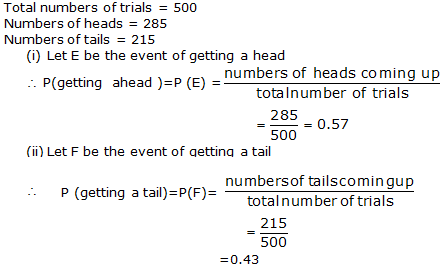Question 2

12 packets of salt, each marked 2 kg, actually contained the following weights (in kg) of salt:

1.950, 2.020, 2.060, 1.980, 2.030, 1.970,

2.040, 1.990, 1.985, 2.025, 2.000, 1.980.

Out of these packets, one packet is chosen at random.

What is the probability that the chosen packet contains more than 2 kg of salt?

Solution 2

Total number of salt packets = 12

Number of packets containing more than 2 kg of salt = 5

Therefore,

Probability that the chosen packet contains more than 2 kg of saltQuestion 3

In a cricket match, a batsman hits a boundary 6 times out of 30 balls he plays. Find the probability that he did not hit a boundary.

Solution 3

Total number of ball played = 30

Number of times boundary was hit = 6

Number of times boundary was not hit = 30 - 6 = 24

Therefore,

Probability that the batsman did not hit the boundaryQuestion 4

An organisation selected 2400 families at random and surveyed them to determine a relationship between the income level and the number of vehicles in a family. The information gathered is listed in the table below:

 Monthly income (in Rs.) Number of vehicles per family 0 1 2 3 or more Less than Rs.25000 10 160 25 0 Rs.25000 - Rs.30000 0 305 27 2 Rs.30000 - Rs.35000 1 535 29 1 Rs.35000 - Rs.40000 2 469 59 25 Rs.40000 or more 1 579 82 88

Suppose a family is chosen at random. Find the probability that the family chosen is

(i) earning Rs.25000 - Rs.30000 per month and owning exactly 2 vehicles.

(ii) earning Rs.40000 or more per month and owning exactly 1 vehicle.

(iii) earning less than Rs.25000 per month and not owning any vehicle.

(iv) earning Rs.35000 - Rs.40000 per month and owning 2 or more vehicles.

(v) owning not more than 1 vehicle.

Solution 4Question 5

The table given below shows the mark obtained by 30 students in a test.

 Marks (Class interval) 1 - 10 11 - 20 21 - 30 31 - 40 41 - 50 Number of students (Frequency) 7 10 6 4 3

Out of these students, one is chosen at random. What is the probability that the marks of the chosen student

(i) are 30 or less?

(ii) are 31 or more?

(iii) lie in the interval 21-30?

Solution 5Question 6

The table given shows the ages of 75 teachers in a school.

 Age (in years) 18 - 29 30 - 39 40 - 49 50 - 59 Number of teachers 3 27 37 8

A teacher from this school is chosen at random. What is probability that the selected teacher is

(i) 40 or more than 40 years old?

(ii) of an age lying between 30-39 years (including both)?

(iii) 18 years or more and 49 years or less?

(iv) 18 years or more old?

(v) above 60 years of age?

NOTE Here 18 - 29 means 18 or more but less than or equal to 29.

Solution 6Question 7

Following are the ages (in years) of 360 patients, getting medical treatment in a hospital:

 Age (in years) 10 - 20 20 - 30 30 - 40 40 - 50 50 - 60 60 - 70 Number of patients 90 50 60 80 50 30

One of the patients is selected at random.

What is probability that his age is

(i) 30 years or more but less than 40 years?

(ii) 50 years or more but less than 70 years?

(iii) 10 years or more but less than 40 years?

(iv) 10 years or more?

(v) less than 10 years?

Solution 7Question 8

The marks obtained by 90 students of a school in mathematics out of 100 are given as under:

 Marks 0-20 20-30 30-40 40-50 50-60 60-70 70 and above No. of students 7 8 12 25 19 10 9

From these students, a student is chosen at random.

What is the probability that the chosen student

(i) get 20% or less marks? (ii) get 60% or more marks?

Solution 8Question 9

It is known that a box of 800 electric bulbs contains 36 defective bulbs. One bulb is taken at random out of the box. What is probability that the bulb chosen is nondefective?

Solution 9

Total number of electric bulbs = 800

Number of defective bulbs = 36

Number of non-defective bulbs = 800 - 36 = 764

Hence, probability that the bulb chosen is non-defectiveQuestion 10

Fill in the blanks.

(i) Probability of an impossible event = ..... .

(ii) Probability of a sure event = ..... .

(iii) Let E be an event. Then, P(not E) = ..... .

(iv) P(E) + P(not E) = ..... .

(v) ..... ≤ P(E) ≤ .... .

Solution 10

Fill in the blanks.

(i) Probability of an impossible event = 0

(ii) Probability of a sure event = 1

(iii) Let E be an event. Then, P(not E) = 1 - P(E)

(iv) P(E) + P(not E) = 1

(v) 0 ≤ P(E) ≤ 1

Question 11

Two coin are tossed 400 times and we get

When two coins are tossed at random, what is the probability of getting

Solution 11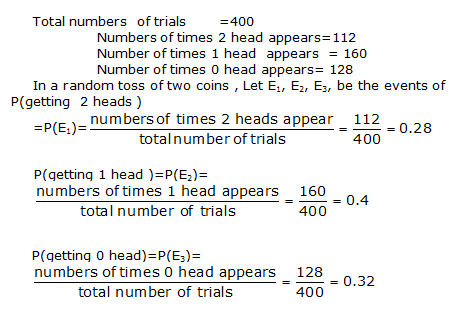Question 12

Three coins are tossed 200 times and we get

When three coins are tossed at random, what is the probability of getting

Solution 12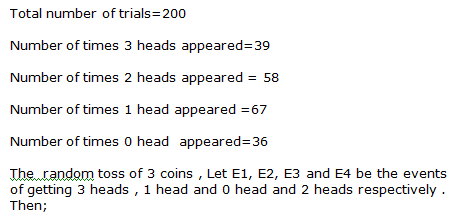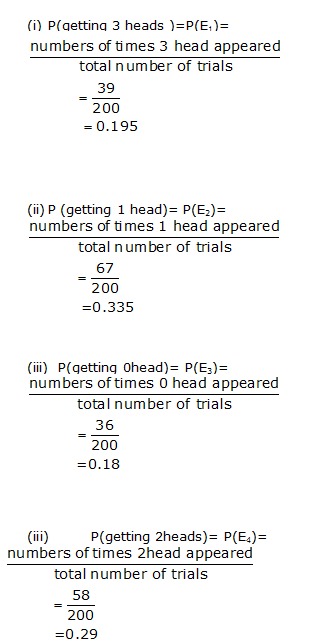Question 13

A die is thrown 300 times and the outcomes are noted as given below:

 Outcome 1 2 3 4 5 6 frequency 60 72 54 42 39 33

When a die is thrown at random, what is the probability of getting a

(i) 3? (ii) 6? (iii) 5? (iv)1?

Solution 13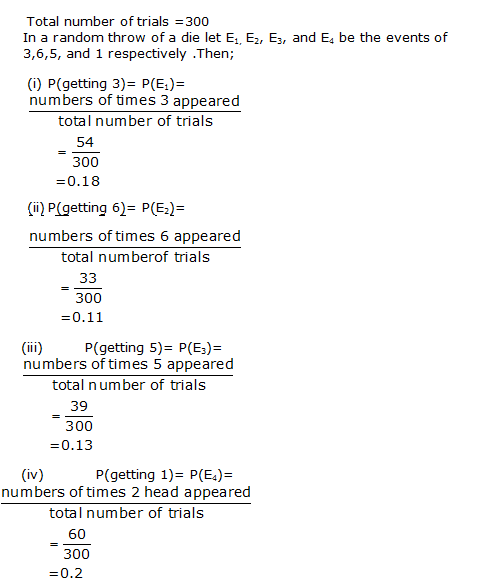Question 14

In a survey of 200 ladies, it was found that 142 like coffee, while 58 dislike it.

Find the probability that a lady chosen at random

(i) Likes coffee (ii) dislikes coffee

Solution 14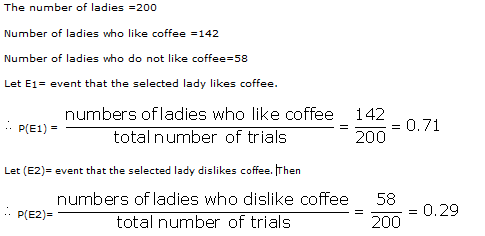Question 15

The percentages of marks obtained by a student in six unit tests are given below :

 Unit test I II III IV V VI Percentageof marksobtained 53 72 28 46 67 59

A unit test is selected at random. What is the probability that the student gets more than 60% marks in the test?

Solution 15

Number of tests in which he gets more than60% marks =2

Total numbers of tests =6Required probabilityQuestion 16

On a particular day, at a crossing in a city, the various types of 240 vehicles going past during a time interval were observed as under:

 Type of vehicles Two-wheelers Three-wheelers Four -wheelers Frequency 84 68 88

Out of these vehicles, one is chosen at random. What is the probability that the chosen vehicle is a two-wheeler?

Solution 16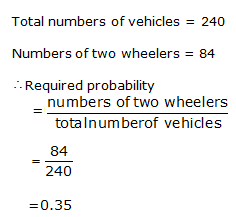Question 17

On one page of a telephone directory, there are 200 phone numbers. The frequency distribution of their units digits is given below:

 Units digit 0 1 2 3 4 5 6 7 8 9 Frequency 19 22 23 19 21 24 23 18 16 15

One of the numbers is chosen at random from the page. What is the probability that the units digit of the chosen number is (i) 5? (ii) 8?

Solution 17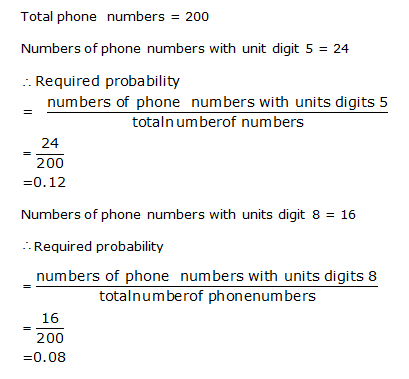Question 18

The following table shows the blood groups of 40 students of the class.

 Blood Group A B O AB Number of students 11 9 14 6

One student of the class is chosen at random. What is the probability that the chosn student has blood group

(i) O? (ii) AB?

Solution 18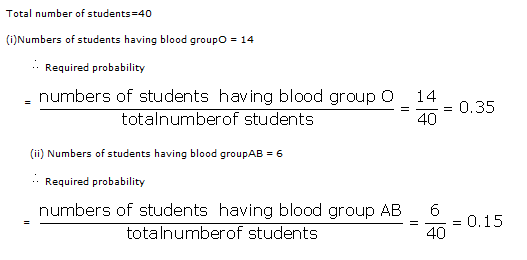## Chapter 19 - Probability Exercise MCQ

Question 1

In a sample survey of 645 people, it was found that 516 people have a high school certificate. If a person is chosen at random, what is the probability that he/she has a high school certificate?

(a)(b)(c)(d)Solution 1

Correct option: (d)

Total number of people = 645

Number of people having high school certificate = 516Question 2

In a cricket match, a batsman hits a boundary 6 times out of 30 balls he plays. What is the probability that in given throw, the ball does not hit the boundary?Solution 2Question 3

A bag contains 16 cards bearing number 1, 2, 3,…, 16 respectively. One card is chosen at random. What is the probability that the chosen card bears a number which is divisible by 3?Solution 3Question 4

A bag contains 5 red, 8 black and 7 white balls. One ball is chosen at random. What is the probability that the chosen ball is blackSolution 4Question 5

In 65 throws of a die, the outcomes were noted as under:

 Outcomes 1 2 3 4 5 6 Number of times 8 10 12 16 9 10

A die is thrown at random. What is probability of getting a prime numberSolution 5Question 6

In 50 throws of a die, the outcomes were notes as under:

 Outcome 1 2 3 4 5 6 Number of times 8 9 6 7 12 8

A die is thrown at random. What is the probability of getting an even number?Solution 6Question 7

The table given below shows the month of birth of 36 students of a class :

 Month of birth Jan Feb March April May June July Aug Sept Oct Nov Dec No.of students 4 3 5 0 1 6 1 3 4 3 4 2

A student is chosen at random from the class. What is the probability that the chosen student was born in October?Solution 7Question 8

Two coins are tossed simultaneously 600 times to get

If two coins are tossed at random, what is the probability of getting at least one head?

(a)(b)(c)(d)Solution 8

Correct option: (c)

Total number of outcomes = 600Question 9

In a medical examination of students of a class, the following blood groups are recorded:

 Blood group A B AB O Number of students 11 15 8 6

From this class, a student is chosen at random. What is the probability that the chosen student has blood group AB?

(a)(b)(c)(d)Solution 9

Correct option: (c)

Total number of students = 11 + 15 + 8 + 6 = 40

Number of students having blood group AB = 8Question 10

80 bulbs are selected at random from a lot and their lifetime in hours is recorded as under.

 Lifetime (in hours) 300 500 700 900 1100 Frequency 10 12 23 25 10

One bulb is selected at random from the lot. What is the probability that its life is 1150 hours?

(a)(b)(c) 1

(d) 0

Solution 10

Correct option: (d)

Total number of bulbs = 80

Number of bulbs having life of 1150 hours = 0

Required probability = 0

Question 11

In a survey of 364 children aged 19 - 36 months, it was found that 91 liked to eat potato chips. If a child is selected at random, the probability that he/she does not like to eat potato chips is

(a)(b)(c)(d)Solution 11

Correct option: (c)

Total number of children = 364

Number of children who like to eat potato chips = 91

Number of children who do not like to eat potato chips = 364 - 91 = 273Question 12

Two coins are tossed 1000 times and the outcomes are recorded as given below:

 Number of heads 2 1 0 Frequency 200 550 250

Now, if two coins are tossed at random, what is the probability of getting at most one head?

(a)(b)(c)(d)Solution 12

Correct option: (b)

Total number of outcomes = 1000Question 13

80 bulbs are selected at random from a lot and their lifetime in hours is recorded as under.

 Lifetime (in hours) 300 500 700 900 1100 Frequency 10 12 23 25 10

One bulb is selected at random from the lot. What is the probability that selected bulb has a life more than 500 hours?

(a)(b)(c)(d)Solution 13

Correct option: (b)

Total number of bulbs = 80Question 14

To know the opinion of the students about the subject Sanskrit, a survey of 200 students was conducted. The data is recorded as under.

 Opinion like dislike Number of students 135 65

What is the probability that a student chosen at random does not like it?

(a)(b)(c)(d)Solution 14

Correct option: (c)

Total number of students = 200

Number of students who does not like Sanskrit = 65Question 15

A coin is tossed 60 times and the tail appears 35 times. What is the probability of getting a head?Solution 15Question 16

It is given that the probability of winning a game is 0.7. What is the probability of losing the game?

(a) 0.8

(b) 0.3

(c) 0.35

(d) 0.15

Solution 16CONTACT :
1800-212-78589:00am - 9:00pm IST all days.
SUBSCRIBE TO TOPPER :
Explore